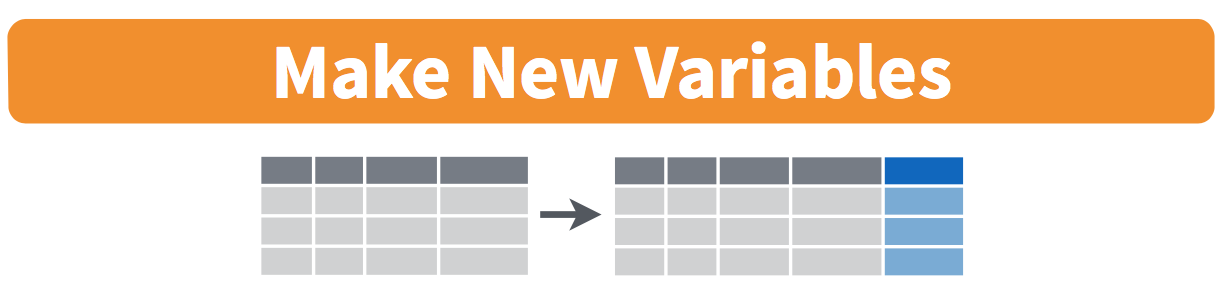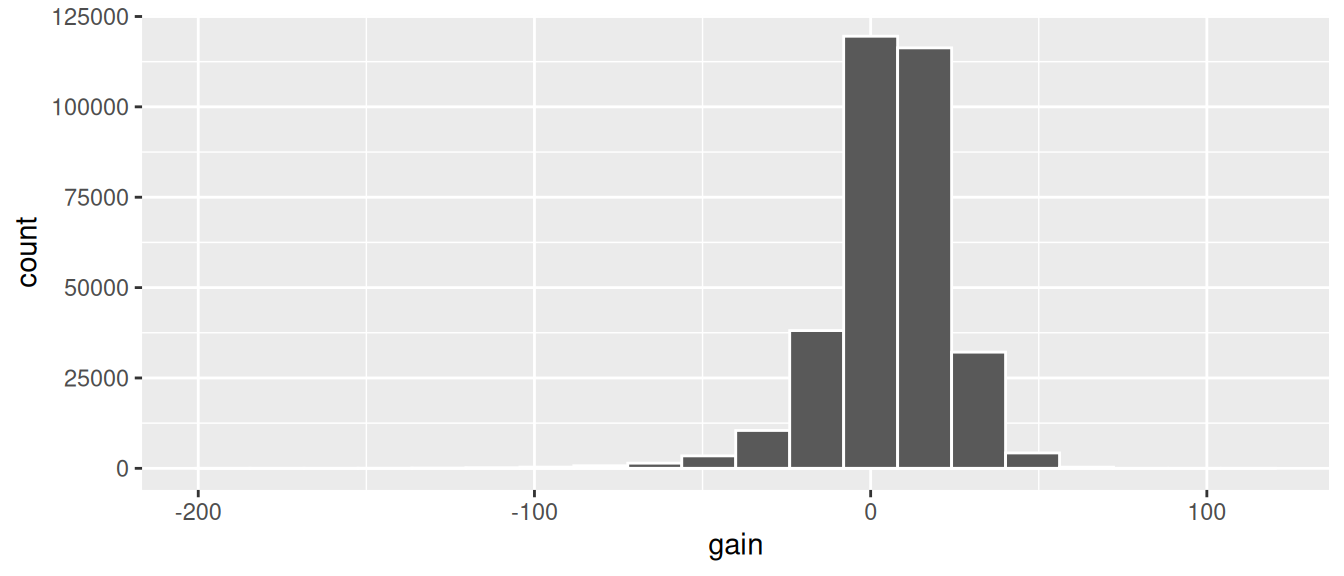## 3.5mutate existing variablesFIGURE 3.5: Diagram of mutate() columns.

Another common transformation of data is to create/compute new variables based on existing ones. For example, say you are more comfortable thinking of temperature in degrees Celsius (°C) instead of degrees Fahrenheit (°F). The formula to convert temperatures from °F to °C is

$\text{temp in C} = \frac{\text{temp in F} - 32}{1.8}$

We can apply this formula to the temp variable using the mutate() function from the dplyr package, which takes existing variables and mutates them to create new ones.

weather <- weather %>%
mutate(temp_in_C = (temp - 32) / 1.8)

In this code, we mutate() the weather data frame by creating a new variable temp_in_C = (temp - 32) / 1.8 and then overwrite the original weather data frame. Why did we overwrite the data frame weather, instead of assigning the result to a new data frame like weather_new? As a rough rule of thumb, as long as you are not losing original information that you might need later, it’s acceptable practice to overwrite existing data frames with updated ones, as we did here. On the other hand, why did we not overwrite the variable temp, but instead created a new variable called temp_in_C? Because if we did this, we would have erased the original information contained in temp of temperatures in Fahrenheit that may still be valuable to us.

Let’s now compute monthly average temperatures in both °F and °C using the group_by() and summarize() code we saw in Section 3.4:

summary_monthly_temp <- weather %>%
group_by(month) %>%
summarize(mean_temp_in_F = mean(temp, na.rm = TRUE),
mean_temp_in_C = mean(temp_in_C, na.rm = TRUE))
summary_monthly_temp
# A tibble: 12 x 3
month mean_temp_in_F mean_temp_in_C
<int>          <dbl>          <dbl>
1     1           35.6           2.02
2     2           34.3           1.26
3     3           39.9           4.38
4     4           51.7          11.0
5     5           61.8          16.6
6     6           72.2          22.3
7     7           80.1          26.7
8     8           74.5          23.6
9     9           67.4          19.7
10    10           60.1          15.6
11    11           45.0           7.22
12    12           38.4           3.58

Let’s consider another example. Passengers are often frustrated when their flight departs late, but aren’t as annoyed if, in the end, pilots can make up some time during the flight. This is known in the airline industry as gain, and we will create this variable using the mutate() function:

flights <- flights %>%
mutate(gain = dep_delay - arr_delay)

Let’s take a look at only the dep_delay, arr_delay, and the resulting gain variables for the first 5 rows in our updated flights data frame in Table 3.1.

TABLE 3.1: First five rows of departure/arrival delay and gain variables
dep_delay arr_delay gain
2 11 -9
4 20 -16
2 33 -31
-1 -18 17
-6 -25 19

The flight in the first row departed 2 minutes late but arrived 11 minutes late, so its “gained time in the air” is a loss of 9 minutes, hence its gain is 2 - 11 = -9. On the other hand, the flight in the fourth row departed a minute early (dep_delay of -1) but arrived 18 minutes early (arr_delay of -18), so its “gained time in the air” is $$-1 - (-18) = -1 + 18 = 17$$ minutes, hence its gain is +17.

Let’s look at some summary statistics of the gain variable by considering multiple summary functions at once in the same summarize() code:

gain_summary <- flights %>%
summarize(
min = min(gain, na.rm = TRUE),
q1 = quantile(gain, 0.25, na.rm = TRUE),
median = quantile(gain, 0.5, na.rm = TRUE),
q3 = quantile(gain, 0.75, na.rm = TRUE),
max = max(gain, na.rm = TRUE),
mean = mean(gain, na.rm = TRUE),
sd = sd(gain, na.rm = TRUE),
missing = sum(is.na(gain))
)
gain_summary
# A tibble: 1 x 8
min    q1 median    q3   max  mean    sd missing
<dbl> <dbl>  <dbl> <dbl> <dbl> <dbl> <dbl>   <int>
1  -196    -3      7    17   109  5.66  18.0    9430

We see for example that the average gain is +5 minutes, while the largest is +109 minutes! However, this code would take some time to type out in practice. We’ll see later on in Subsection 5.1.1 that there is a much more succinct way to compute a variety of common summary statistics: using the skim() function from the skimr package.

Recall from Section 2.5 that since gain is a numerical variable, we can visualize its distribution using a histogram.

ggplot(data = flights, mapping = aes(x = gain)) +
geom_histogram(color = "white", bins = 20)FIGURE 3.6: Histogram of gain variable.

The resulting histogram in Figure 3.6 provides a different perspective on the gain variable than the summary statistics we computed earlier. For example, note that most values of gain are right around 0.

To close out our discussion on the mutate() function to create new variables, note that we can create multiple new variables at once in the same mutate() code. Furthermore, within the same mutate() code we can refer to new variables we just created. As an example, consider the mutate() code Hadley Wickham and Garrett Grolemund show in Chapter 5 of R for Data Science (Grolemund and Wickham 2017):

flights <- flights %>%
mutate(
gain = dep_delay - arr_delay,
hours = air_time / 60,
gain_per_hour = gain / hours
)

Learning check

(LC3.10) What do positive values of the gain variable in flights correspond to? What about negative values? And what about a zero value?

(LC3.11) Could we create the dep_delay and arr_delay columns by simply subtracting dep_time from sched_dep_time and similarly for arrivals? Try the code out and explain any differences between the result and what actually appears in flights.

(LC3.12) What can we say about the distribution of gain? Describe it in a few sentences using the plot and the gain_summary data frame values.

### References

Grolemund, Garrett, and Hadley Wickham. 2017. R for Data Science. First. Sebastopol, CA: O’Reilly Media. https://r4ds.had.co.nz/.